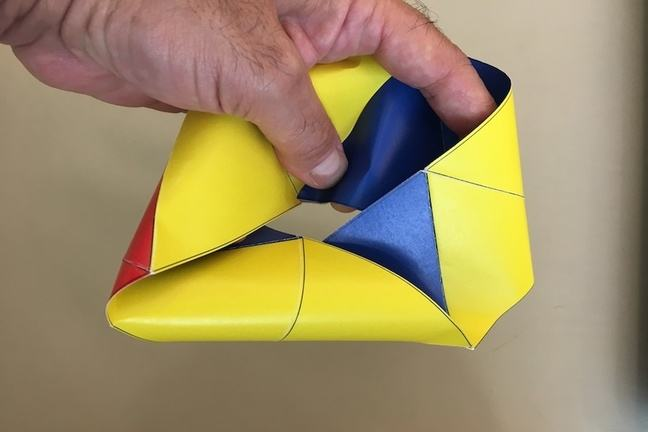## Want to keep learning?

This content is taken from the Davidson Institute of Science Education at the Weizmann Institute of Science's online course, Flexagons and the Math Behind Twisted Paper. Join the course to learn more.
3.5

## Davidson Institute of Science Education at the Weizmann Institute of Science# Cyclic flexagons are Möbius strips!

At first glance, it looks like flexagons and Möbius strips are exact opposites. Möbius strips have one or two sides, depending on the parity of the number of half twists. Flexagons have three or more sides.

In truth, Möbius strips and flexagons are one of the same, at least from a topological point of view. This means that they have exactly the same structure. Take a look at the tri-hexa-flexagon. If you squish it, like I did in the image above, you can see that it is in fact a straight strip of paper, half-twisted three times. In other words, the regular tri-hexa-flexagon is a 3-strip.

Two pioneers in flexagon theory, Conrad and Hartline, found that a cyclic flexagon has the same topological structure as a Möbius band with the same number of half-twists. We’ll refer to Möbius bands that have the same topological structure as a flexagon as its “Möbius band twin”. The number of half-twists in the “Möbius band twin” of a straight strip cyclic flexagon with N faces and n triangles per face is 3N-n. For example, the tri-hexa-flexagon is a cyclic straight strip flexagon with N=3 faces and n=6 triangles on each face. Its “Möbius band twin” has 3N-n=3x3-6=3 half-twists.

This has some very important consequences. In particular, this shows that flexagons constructed whose “Möbius band twin” has an even number of half-twists, should have in theory two independent Tuckerman diagrams. Take the hexa-hexa-flexagon as an example. It is equivalent to the 12-band and so, has an even number of half-twists. We know that performing the V-flex iteratively, transfers us from the regular Tuckerman diagram to the Tuckerman diagram of the completely inverted strip, while exchanging dominant and hidden faces.

### Discussion

Any questions? If so, please post below so that we can answer!